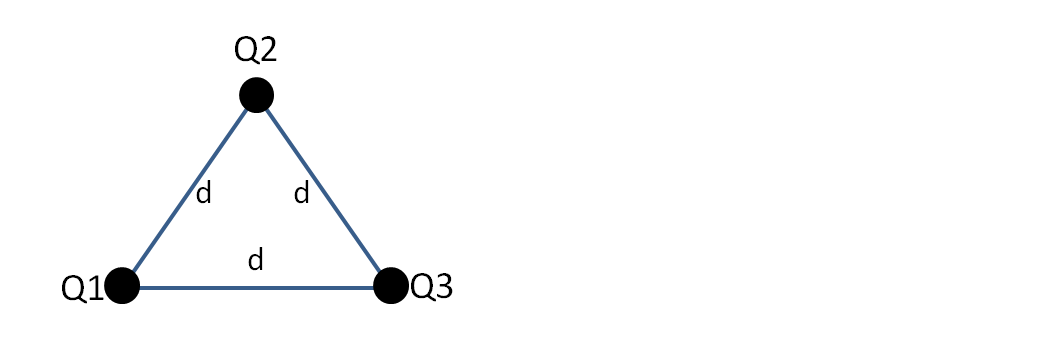# Problem: Three identical point charges, Q = 3μC, are placed at the vertices of an equilateral triangle as shown in the figure. The length of each side of the triangle is d = 0.15m. Determine the magnitude and direction of the total electrostatic force on the charge at the top of the triangle.

🤓 Based on our data, we think this question is relevant for Professor Lewis' class at UNR.

###### Problem Details

Three identical point charges, Q = 3μC, are placed at the vertices of an equilateral triangle as shown in the figure. The length of each side of the triangle is d = 0.15m. Determine the magnitude and direction of the total electrostatic force on the charge at the top of the triangle.What scientific concept do you need to know in order to solve this problem?

Our tutors have indicated that to solve this problem you will need to apply the Coulomb's Law (Electric Force) concept. You can view video lessons to learn Coulomb's Law (Electric Force). Or if you need more Coulomb's Law (Electric Force) practice, you can also practice Coulomb's Law (Electric Force) practice problems.

What is the difficulty of this problem?

Our tutors rated the difficulty ofThree identical point charges, Q = 3μC, are placed at the ve...as medium difficulty.

How long does this problem take to solve?

Our expert Physics tutor, Juan took 6 minutes and 10 seconds to solve this problem. You can follow their steps in the video explanation above.

What professor is this problem relevant for?

Based on our data, we think this problem is relevant for Professor Lewis' class at UNR.